Anzeige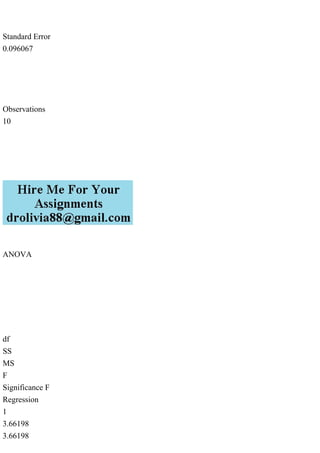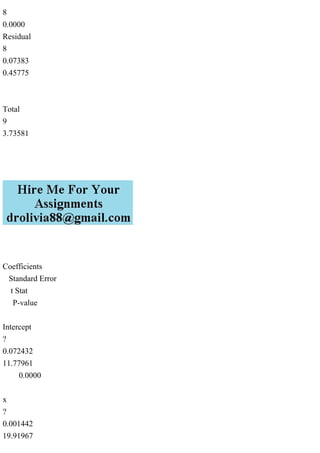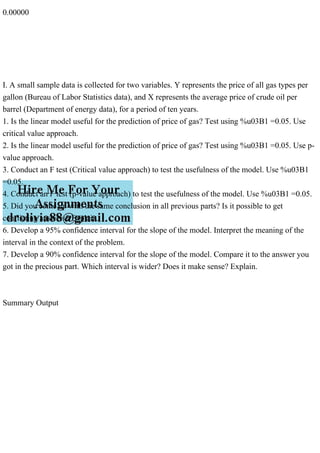Anzeige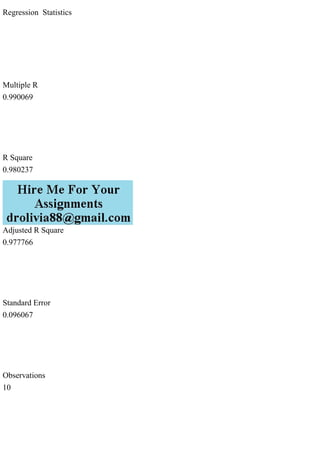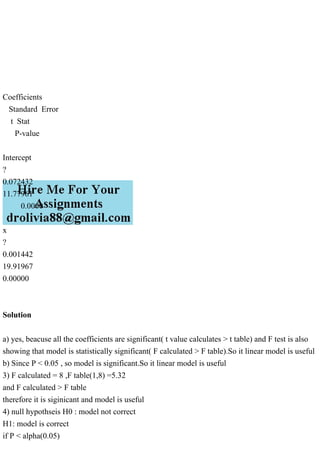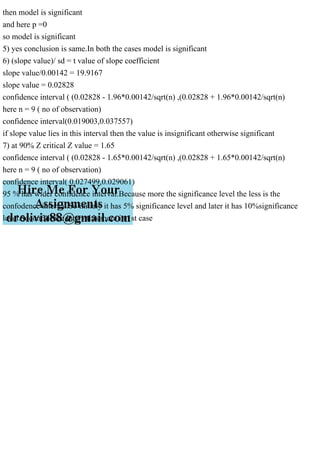Nächste SlideShare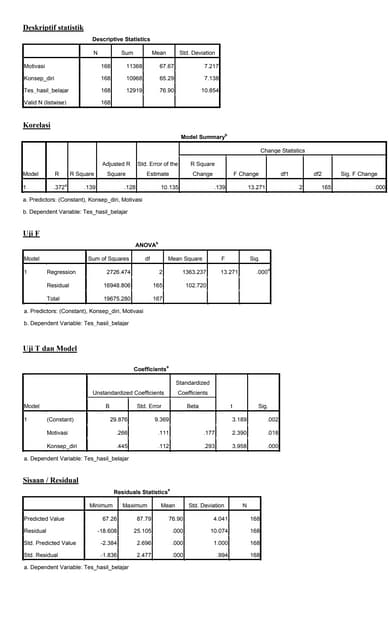Mbak dayani regresi berganda
1 von 8
Anzeige

### Summary OutputRegression Statistics.pdf

1. Summary Output Regression Statistics Multiple R 0.990069 R Square 0.980237 Adjusted R Square 0.977766
2. Standard Error 0.096067 Observations 10 ANOVA df SS MS F Significance F Regression 1 3.66198 3.66198
3. 8 0.0000 Residual 8 0.07383 0.45775 Total 9 3.73581 Coefficients Standard Error t Stat P-value Intercept ? 0.072432 11.77961 0.0000 x ? 0.001442 19.91967
4. 0.00000 I. A small sample data is collected for two variables. Y represents the price of all gas types per gallon (Bureau of Labor Statistics data), and X represents the average price of crude oil per barrel (Department of energy data), for a period of ten years. 1. Is the linear model useful for the prediction of price of gas? Test using %u03B1 =0.05. Use critical value approach. 2. Is the linear model useful for the prediction of price of gas? Test using %u03B1 =0.05. Use p- value approach. 3. Conduct an F test (Critical value approach) to test the usefulness of the model. Use %u03B1 =0.05. 4. Conduct an F test (p-value approach) to test the usefulness of the model. Use %u03B1 =0.05. 5. Did you come up with the same conclusion in all previous parts? Is it possible to get conflicting answers? Explain. 6. Develop a 95% confidence interval for the slope of the model. Interpret the meaning of the interval in the context of the problem. 7. Develop a 90% confidence interval for the slope of the model. Compare it to the answer you got in the precious part. Which interval is wider? Does it make sense? Explain. Summary Output
5. Regression Statistics Multiple R 0.990069 R Square 0.980237 Adjusted R Square 0.977766 Standard Error 0.096067 Observations 10
6. ANOVA df SS MS F Significance F Regression 1 3.66198 3.66198 8 0.0000 Residual 8 0.07383 0.45775 Total 9 3.73581
7. Coefficients Standard Error t Stat P-value Intercept ? 0.072432 11.77961 0.0000 x ? 0.001442 19.91967 0.00000 Solution a) yes, beacuse all the coefficients are significant( t value calculates > t table) and F test is also showing that model is statistically significant( F calculated > F table).So it linear model is useful b) Since P < 0.05 , so model is significant.So it linear model is useful 3) F calculated = 8 ,F table(1,8) =5.32 and F calculated > F table therefore it is siginicant and model is useful 4) null hypothseis H0 : model not correct H1: model is correct if P < alpha(0.05)
8. then model is significant and here p =0 so model is significant 5) yes conclusion is same.In both the cases model is significant 6) (slope value)/ sd = t value of slope coefficient slope value/0.00142 = 19.9167 slope value = 0.02828 confidence interval ( (0.02828 - 1.96*0.00142/sqrt(n) ,(0.02828 + 1.96*0.00142/sqrt(n) here n = 9 ( no of observation) confidence interval(0.019003,0.037557) if slope value lies in this interval then the value is insignificant otherwise significant 7) at 90% Z critical Z value = 1.65 confidence interval ( (0.02828 - 1.65*0.00142/sqrt(n) ,(0.02828 + 1.65*0.00142/sqrt(n) here n = 9 ( no of observation) confidence interval( 0.027499,0.029061) 95 % has wider confidence interval.Because more the significance level the less is the confodence interval.So initialy it has 5% significance level and later it has 10%significance level.Soconfidence interval is more in 1st case
Anzeige